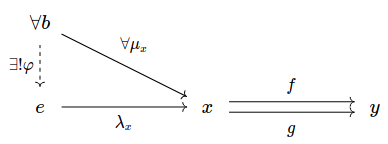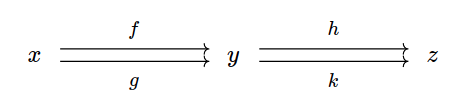# Commutative diagrams

(diff) ← Older revision | Latest revision (diff) | Newer revision → (diff)

## Bound and Free Variables

In category theory, reading commutative diagrams requires an understanding of which variables are bound and which are free. Some authors choose to annotate their diagrams with universal quantifiers like $$\forall x,y,z$$ to indicate the set of free variables, implying the rest are bound.

When such annotations are not included, the diagram alone is not precise enough to decode the intended logical statement. To read a commutative diagram, we must infer the bound and free variables from context.

## When Commutative Diagrams Don't Commute

When encountering commutative diagrams for the first time, many students are told that whenever there is more than one path between two objects in a commutative diagram, the corresponding morphisms must always commute. A notable exception occurs when there is a pair of parallel directed edges between two objects, as in the following equalizer diagram. Without additional context, parallel arrows in a commutative diagram are not necessarily the same, even if they may participate in other commutative paths.This is a typical commutative diagram depicting the universal property of the equalizer of two morphisms $$f, g : x \rightarrow y$$. Here, $$f$$ and $$g$$ are two different paths between the objects $$x$$ and $$y$$, but in fact $$f$$ and $$g$$ are assumed to be distinct morphisms.

For example, consider the diagram below. The statement "this diagram commutes" is equivalent to the statement "$$h \circ f = h \circ g = k \circ f = k \circ g$$". It is not necessarily implied that $$f = g$$ or $$h = k$$.A commutative diagram with three objects (x, y, z). There are two parallel morphisms (f,g) from x to y, and two parallel morphisms (h,k) from y to z.